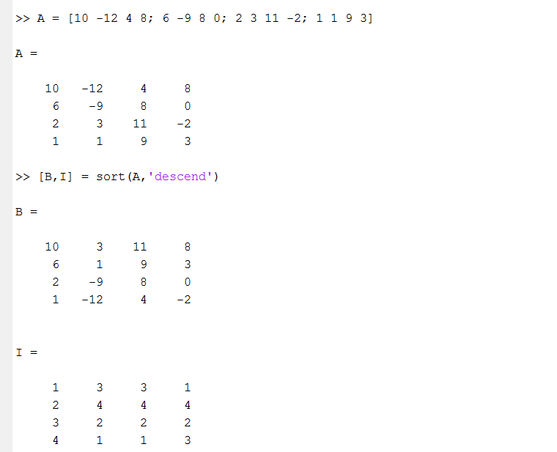World Statistics Day was yesterday, but we’re celebrating all week long! This celebration means acknowledging the impact statistics has on our world. Who is your favorite statistician? Share with us who they are and why they top your favorites list.
Choose Language Hide Translation Bar
Highlighted

## Sort a matrix

All,
Is there a way to achieve functionality similar to what is shown below :

``A = [10 -12 4 8; 6 -9 8 0; 2 3 11 -2; 1 1 9 3]``

Upon sorting, how can i retain the original positions of the elements ?

For e.g. if i wanted to sort each column of the matrix, I can only think of doing the following:

``````for(i = 1, i <= N cols(A), i ++,
t = A[0,i];
t1 = sort descending(t);
);``````

Now, how can I determine the original position of the elements and retain them ?
https://www.mathworks.com/help/matlab/ref/sort.html - In Matlab, there is a second argument to the sort function which returns the index

Similarly in R, you can retain the index. Wondering what is the best way to do that in JMP ?

Best
Uday
1 ACCEPTED SOLUTION

Accepted Solutions
Highlighted

## Re: Sort a matrix

`A = [10 -12 4 8; 6 -9 8 0; 2 3 11 -2; 1 1 9 3];sort matrix = Function( { m }, { t, b=[], i=[] }, For( j = 1, j <= N Cols( m ), j++, t = A[0,j]; b ||= Sort Descending( t ); i ||= Matrix( Reverse( As List( Rank Index( t ) ) ) ); ); Eval List( { b, i } ););{ B, I } = sort matrix( A );Show( A, B, I );`

Learn it once, use it forever!
6 REPLIES 6
Highlighted

## Re: Sort a matrix

Take a look at "Ranking and Sorting" in the "Data Structures, Matrices" section of the Scripting Guide
Jim
Highlighted

## Re: Sort a matrix

@txnelson
I believe you are referring to the content that is also covered here - https://www.jmp.com/support/help/14/ranking-and-sorting.shtml . However, this does not expand on capturing the indices that I am looking for while performing the sort. Hopefully, I am not missing something.

Best
Uday
Highlighted

## Re: Sort a matrix

Um, it is the ranking bit...

Learn it once, use it forever!
Highlighted

## Re: Sort a matrix

@markbailey & @txnelson
I understand that it looks obvious to you that Rank() does what I want, however , here is an example of why I am asking for:

In Matlab, here is what happen when you try to sort a matrix. Apologies for pulling this example from a different platform, I tried to avoid doing that but I can't think of a better way to explain what I am after. So if you look at the values of  "I", it retains the indicesNow, if I wanted to do the exact samething in JMP , is this the way you are recommending me to take, it yes, it doesn't get me the same result. Also, I would appreciate not having to use a loop for something like this

``````A = [10 -12 4 8; 6 -9 8 0; 2 3 11 -2; 1 1 9 3];

for(i = 1 , i <= N Cols(A), i++,
t = A[0,i];
t1 = Sort Descending(t);
ranks = Rank(t);
);``````

Best
Uday
Highlighted

## Re: Sort a matrix

`A = [10 -12 4 8; 6 -9 8 0; 2 3 11 -2; 1 1 9 3];sort matrix = Function( { m }, { t, b=[], i=[] }, For( j = 1, j <= N Cols( m ), j++, t = A[0,j]; b ||= Sort Descending( t ); i ||= Matrix( Reverse( As List( Rank Index( t ) ) ) ); ); Eval List( { b, i } ););{ B, I } = sort matrix( A );Show( A, B, I );`

Learn it once, use it forever!
Highlighted

## Re: Sort a matrix

@markbailey
Thank you. I will submit a request to the wish list to provide a canned function for this.  I hope in the future, JMP will come up with a way to not have to loop through the matrix. I would love to see the apply() function family from R introduced into JMP.

Best
Uday
Article Labels

There are no labels assigned to this post.